Next: 3-d problems Up: Poisson's equation Previous: An example solution of

## Example 2-d electrostatic calculation

Let us perform an example 2-d electrostatic calculation. Consider a charged wire running parallel to the axis of a uniform, hollow, rectangular, conducting channel. Suppose that the vertices of the channel lie at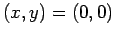,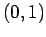,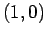, and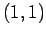. Suppose, further, that the wire carries a uniform charge per unit length of magnitude unity. The electric potential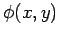inside the channel satisfies [see Eq. (110)]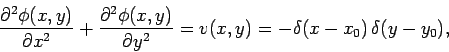(185)

where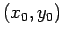are the coordinates of the wire. Here, we have conveniently normalized our units such that the factor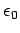is absorbed into the normalization. Assuming that the box is grounded, the potential is subject to the Dirichlet boundary conditions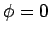at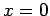,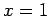,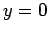, and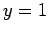. We require the solution in the region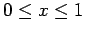and.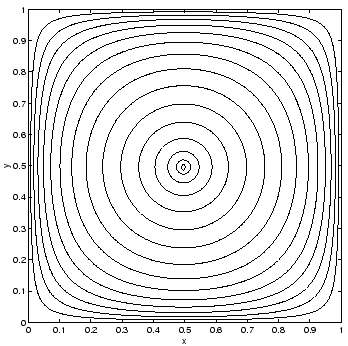Note that when discretizing Eq. (185) the right-hand side becomes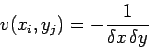(186)

on the grid-point closest to the wire, with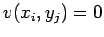on the remaining grid-points. Here,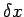and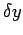are the grid spacings in the- and- directions, respectively.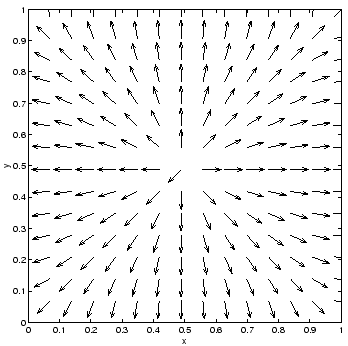Figures 67 and 68 show the electric potentialand electric field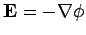generated by a wire placed at the center of the channel: i.e.,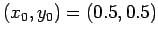. The calculation was performed with the previously listed 2-d Poisson solver using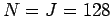.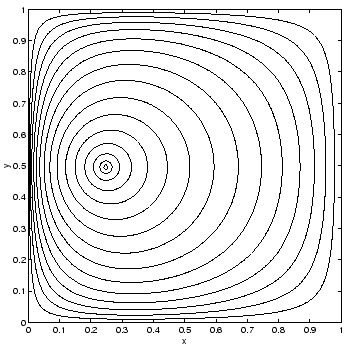Figures 69 and 70 show the electric potentialand electric fieldgenerated by a wire offset from the center of the channel: i.e.,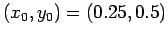. The calculation was performed with the previously listed 2-d Poisson solver using.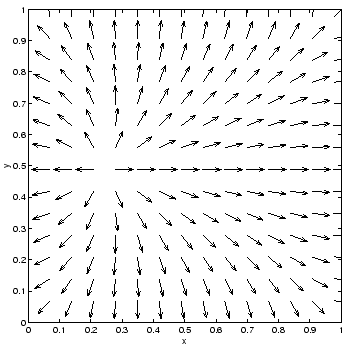Next: 3-d problems Up: Poisson's equation Previous: An example solution of
Richard Fitzpatrick 2006-03-29